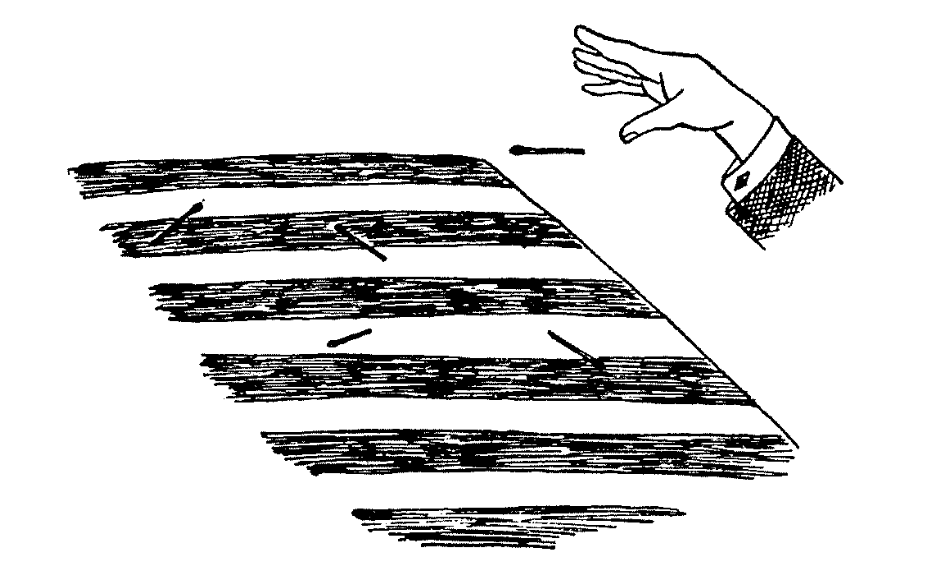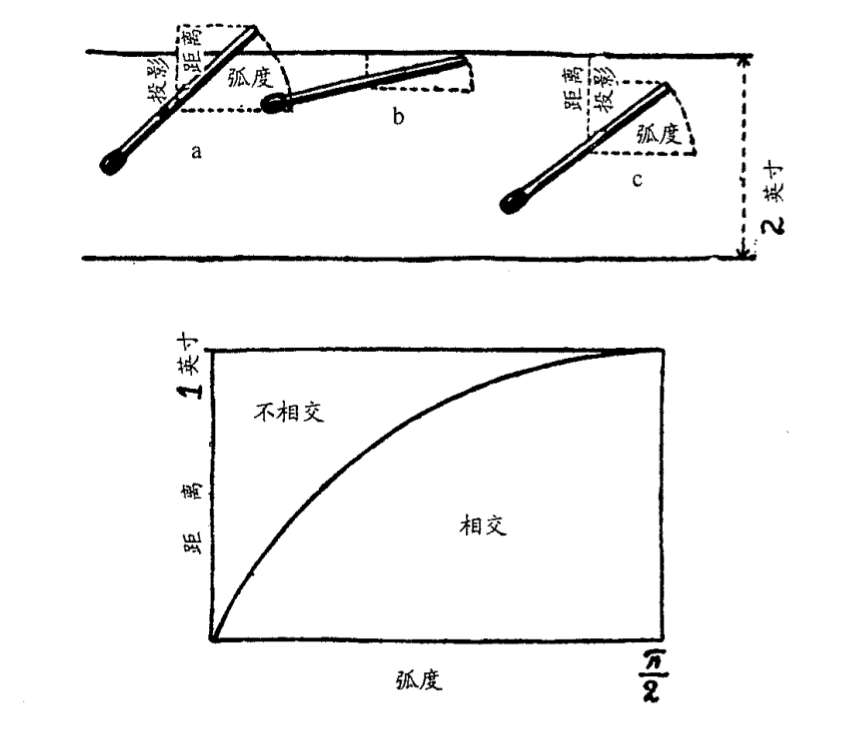## 质数的分布

1 ~ 100 26 0.260 0.217 20
1 ~ 1000 168 0.168 0.145 16
1 ~ ${10^6}$ 78498 0.078498 0.072382 8
1 ~ ${10^9}$ 50847478 0.050847478 0.048254942 5

## 勾股定理和无理数

$$p^2=2q^2$$我们假设 ${p \over q}$ 已经是不可约的，因为分子和分母的任意公因子在一开始就可约去。由于2是上式等号右边的一个因子，所以左边的 $p^2$ 是偶数，故 $p$ 本身是偶数，因为奇数的平方只能是奇数，于是我们可以写出 $p=2r$，其中 $r$ 为整数。这时上式变为
$$4r^2=2q^2，或 2r^2=q^2$$由于 2 是左边的因子，则 $q$ 必须为偶数，这样一来 $p$ 和 $q$ 同时可以被 2 整除，和 $p$, $q$ 没有公因子的假设矛盾，因此 $p^2=2q^2$ 不成立，且 $x$ 不能是有理数。

## 神秘的圆周率

18 世纪，数学领域开始出现一个新的分支，概率。有个法国科学家，提了一个概率问题，为了实验这个概率问题，需要有一面美国国旗，即红白条相 间的旗子。如果没有这种旗子，在一大张纸上画上若干道等距的 平行线也可以。再要一盒火柴——什么火柴都成，只要短于平行线间的距离就可以。现在把旗子铺在桌子上，扔出一根火柴，让它落在旗子上，如下图 1。它可能完全落在一条带子里，也可能压在两条带子上。 这两种情况发生的机会各为多大呢?• 《什么是数学》
• 《从一到无穷大》